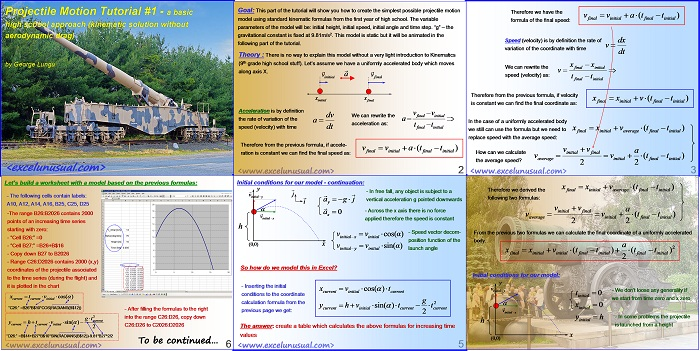# 2D Projectile Motion Tutorial #1

This part of the tutorial will show you how to create the simplest possible projectile motion model using standard kinematic formulas from the first year of high school.

The variable parameters of the model will be: initial height, initial speed and initial angle and time step. “g” – the gravitational constant will be set at 9.81 m/s^2.

This model is a static one and it will be animated in the following part of the tutorial.[sociallocker][/sociallocker]

## Projectile Motion Tutorial #1 – a basic high school approach (kinematic solution without aerodynamic drag)

by George Lungu

### Goal:

This part of the tutorial will show you how to create the simplest possible projectile motion
model using standard kinematic formulas from the first year of high school. The variable
parameters of the model will be: initial height, initial speed, initial angle and time step. “g” – the
gravitational constant is fixed at 9.81m/s2. This model is static but it will be animated in the
following part of the tutorial.

### Theory :

There is no way to explain this model without a very light introduction to Kinematics
(9th grade high school stuff). Let’s assume we have a uniformly accelerated body which moves
along axis X.

initial final

Acceleration is by definition the rate of variation of the velocity in time.

We can rewrite the acceleration as:

speed (velocity) with time dt final initial
Therefore from the previous formula, if acceleration is constant we can find the final speed as:

final initial

<www.excelunusual.com> 2

#### Therefore we have the formula of the final speed:

Speed(velocity) is by definition the rate of variation of the coordinate with time

We can rewrite the speed (velocity) as:

Therefore from the previous formula, if velocity is constant we can find the final coordinate as:

In the case of a uniformly accelerated body we still can use the formula, but we need to replace
speed with the average speed:

How can we calculate the average speed?

<www.excelunusual.com> 3

Therefore we derived the following two formulas:

From the previous two formulas we can calculate the final coordinate of a uniformly accelerated
body:

### Initial conditions for our model:

– We don’t loose any generality if we start from time zero and x zero.

– In some problems the projectile is launched from a height:

#### Initial conditions for our model – continuation:

– In free fall, any object is subject to a vertical acceleration g pointed downwards.

– Across the x axis there is no force applied therefore the speed is constant.

– Speed vector decomposition function of the launch angle:

### So how do we model this in Excel?

– Inserting the initial conditions to the coordinate
calculation formula from the previous page we get:

The answer: create a table which calculates the above formulas for increasing time values.

<www.excelunusual.com> 5

#### Let’s build a worksheet with a model based on the previous formulas:

– The following cells contain labels: A10, A12, A14, A16, B25, C25, D25

-The range B26:B2026 contains 2000 points of an increasing time series starting with zero:

– “Cell B26:” =0

– “Cell B27:” =B26+B\$16

– Copy down B27 to B2026

– Range C26:D2026 contains 2000 (x,y) coordinates of the projectile associated to the time series
(during the flight) and it is plotted in the chart.